# Using the Other Formulas to Find the Equation of a Straight Line From Two Points

## A Little Bit of NonsenseMeet Edward the Echidna. Being a very mathematically-minded monotreme, he has decided to model the growth of the termite mounds in his neighbourhood. He has observed that the height of termite mounds grows linearly as a function of the base radius, and that harvesting them is most efficient when their base radius measures 20 snouts. A "snout" is a standard echidna unit of measurement. He wants to know what the height of a newly discovered termite mound will be when the base radius is 20 snouts. After several weeks of observation, he knows that, when the base radius measured 3 snouts, the height of the mound was 4 snouts, and when the base radius measured 4 snouts, the height of the mound was 5 snouts.

Putting this all together, we can see that Edward is looking for the equation of a straight line which passes through the points $(3,4)$ and $(4,5)$. He will then need to substitute in the value $\text{radius} = 20$ to find the height of the termite mound. But how can he find this equation? We know how to find the equation if we know the gradient and the $y$-intercept of the line, or the gradient and a point on the line. How can we find it if we only know two points on the line?

## Cartesian Coordinates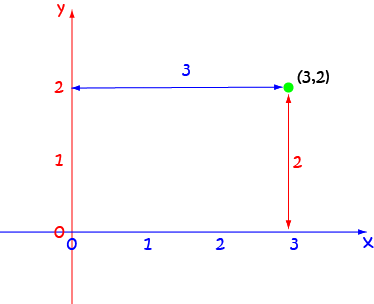What do those pairs of numbers in Edward's story actually mean? They are Cartesian coordinates and they give us a way of specifying the position of a point in the $xy$-plane. The first number is called the $x$-coordinate. It tells you how far across the plane the point lies. The second number is called the $y$-coordinate, and it tells you how far up the plane the point lies. The picture shows the position of the point with Cartesian coordinates $(3,2)$ in the $xy$-plane. It is located 3 units to the right and two units up from the axes.

If you like memorising lots of different equations, then there is a version of the equation of a straight line that will take you directly to the answer without any further calculation. If this sounds appealing, check out the article on the equation of a straight line from two points.

However, if you're like me and really don't like memorising lots of different equations for the same thing, this article shows you how to use the other two forms of the equation of a straight line that we know about to do the same job. Both of them require a calculation of the gradient, so we'd better do a quick recap on how you find that.

## Finding the Gradient of a Line from Two Points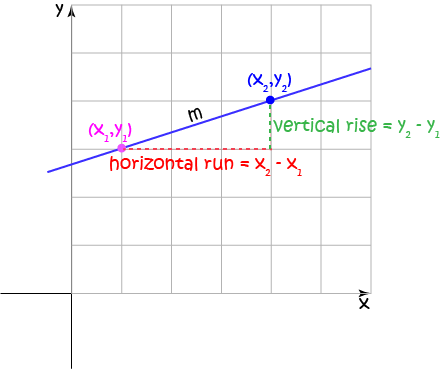What if we only know the two points $(x_1,y_1)$ and $(x_2,y_2)$, and not the gradient of a line? Well, we can work out what the gradient is. Let's hop to it (do echidnas hop? Maybe "scurry" is a better word.):

$m = \text{gradient} = \dfrac{\text{change in y}}{\text{change in x}} = \dfrac{y_2 - y_1}{x_2 - x_1}.$

### Example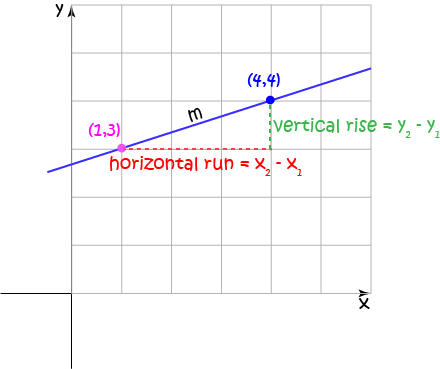Let's find the gradient of the line segment joining the two points $(1,3)$ and $(4,4)$. It is

$m =\dfrac{y_2 - y_1}{x_2 - x_1} = \dfrac{4 - 3}{4 - 1} = \dfrac{1}{3}.$

## Using the Point-Gradient Form of the Equation

If you know the gradient $m$ of a straight line and one point $(x_1,y_1)$ that it passes through, then you can use that information to find the point-gradient equation of the straight line as follows. It is:

$y - y_1 = m (x - x_1).$
We can use this equation to find the equation of a straight line through two points $(x_1,y_2)$ and $(x_2,y_2)$. Here are the steps we need to follow:
1. Find the gradient using $m =\dfrac{y_2 - y_1}{x_2 - x_1}$
2. Choose one of the points (doesn't matter which) and plug it, and the gradient, into the equation $y - y_1 = m (x - x_1).$
3. Massage your equation into the form $y = mx + b$.

Let's try this equation out on a couple of examples.

### Example 1

Find the equation of the line through the points $(0,1)$ and $(-2, -2)$.

Solution:

Set $(x_1,y_1) = (0,1)$ and $(x_2,y_2) = (-2,-2)$. Then

\begin{align*} m = \dfrac{y_2 - y_1}{x_2 - x_1} &= \dfrac{-2 - 1}{-2 - 0}\\ &= \dfrac{-3}{-2}\\ &= \dfrac{3}{2} \end{align*}
and, if we choose the point $(0,1)$, the equation of our line is
\begin{align*} y - 1 &= \dfrac{3}{2} (x - 0)\\ y - 1 &= \dfrac{3}{2}\;x \\ y &= \dfrac{3}{2}\;x + 1 \end{align*}
So the graph looks like this: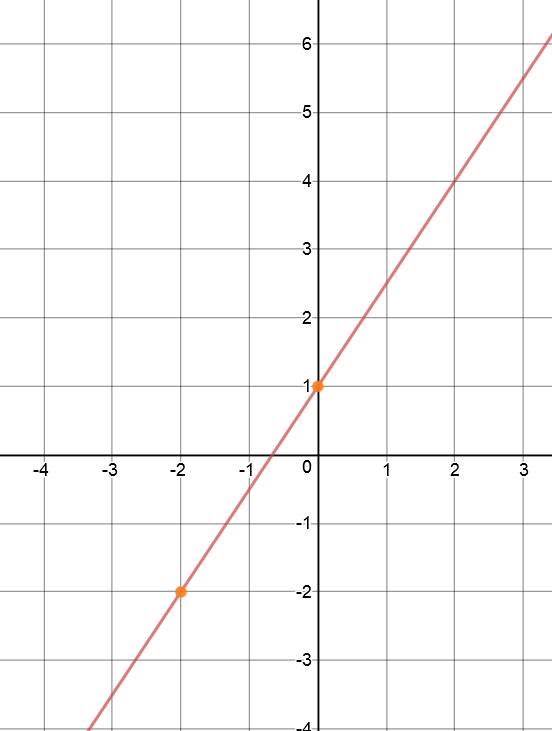### Example 2

Find the equation of the line through the points $(-4,-1)$ and $(-2, 0)$.

Solution:

Set $(x_1,y_1) = (-4,-1)$ and $(x_2,y_2) = (-2,0)$. Then

\begin{align*} m = \dfrac{y_2 - y_1}{x_2 - x_1} &= \dfrac{0 - (-1)}{-2 - (-4)}\\ &= \dfrac{1}{2} \end{align*}
and, if we choose the point $(-2,0)$, the equation of our line is
\begin{align*} y - 0 &= \dfrac{1}{2} (x - (-2))\\ y &= \dfrac{1}{2}\;x + \dfrac{1}{2}(2)\\ y &= \dfrac{1}{2}\;x + 1 \end{align*}
So the graph looks like this: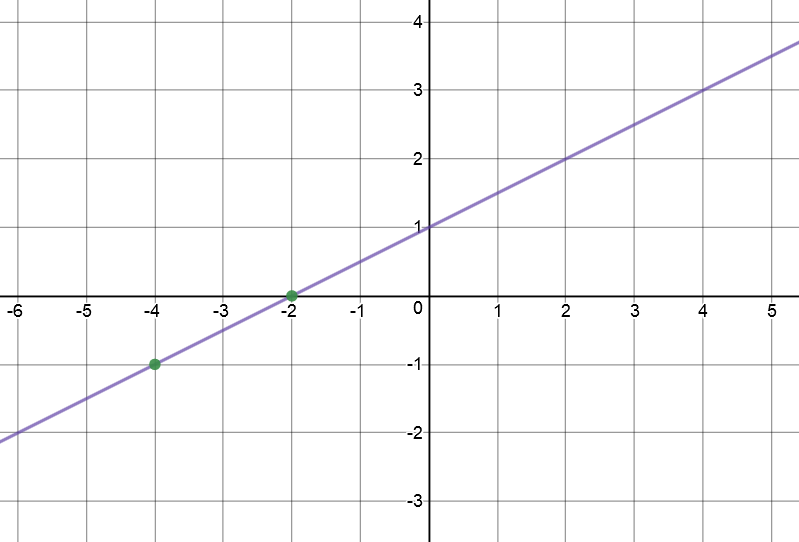## Using the Gradient-Intercept Equation of a Straight Line

I'm allergic to memorising too many formulas, and I think that it's enough to memorise $y = mx + b$ as the equation of a straight line.

Here's what I do:

1. Find the gradient using $m =\dfrac{y_2 - y_1}{x_2 - x_1}$.
2. Plug one of the points into the equation $y = mx + b$ (doesn't matter which) and solve to find $b$.
3. Write down the equation of the line in the form $y = mx + b$.

Let's try an example, and then look at Edward's problem.

### Example 3

Find the equation of the line through the points $(2,-4)$ and $(-4, 8)$.

Solution:

Set $(x_1,y_1) = (2,-4)$ and $(x_2,y_2) = (-4,8)$. Then

\begin{align*} m = \dfrac{y_2 - y_1}{x_2 - x_1} &= \dfrac{8 - (-4)}{-4 - 2}\\ &= \dfrac{12}{-6}\\ &= -2. \end{align*}
So, the equation is $y = -2x + b$, and we have to find $b$. Plug one of the points, say $(2,-4)$, into the equation and solve for $b$:
\begin{align*} y &= -2x + b\\ -4 &= -2(2) + b\\ -4 &= -4 + b\\ 0 &= b \end{align*}
So, the equation of the line is $y = -2x$, and the graph looks like this: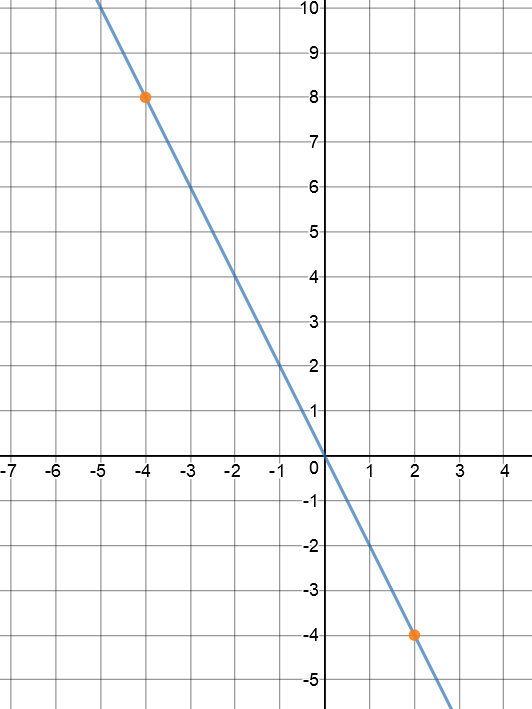### Now It's Time to Solve Edward's Problem

Do you remember that Edward wanted to find the height of his termite hill when its base radius measured 20 snouts? He knew that the height was 4 snouts when the base radius was 3 snouts and the height was 6 snouts when the base radius was 5 snouts. The height grew linearly with respect to the base radius, so he was looking for the equation of a straight line through the points $(3,4)$ and $(5,6)$. Setting $(\text{radius}_1,\text{height}_1) = (3,4)$ and $(\text{radius}_2,\text{height}_2) = (5,6)$, we obtain

\begin{align*} m &= \dfrac{\text{height}_2- \text{height}_1}{\text{radius}_2 - \text{radius}_1}\\ &= \dfrac{6 - 4}{5 - 3}\\ &= \dfrac{2}{2}\\ &= 1 \end{align*}
Then the equation is $\text{height} = \text{radius} + b$. Plug one of the points into this equation, and solve to find $b$:
\begin{align*} \text{height} &= \text{radius} + b\\ 4 &= 3 + b\\ 1 &= b \end{align*}
So, the equation is $\text{height} = \text{radius} + 1$, and the graph looks like this: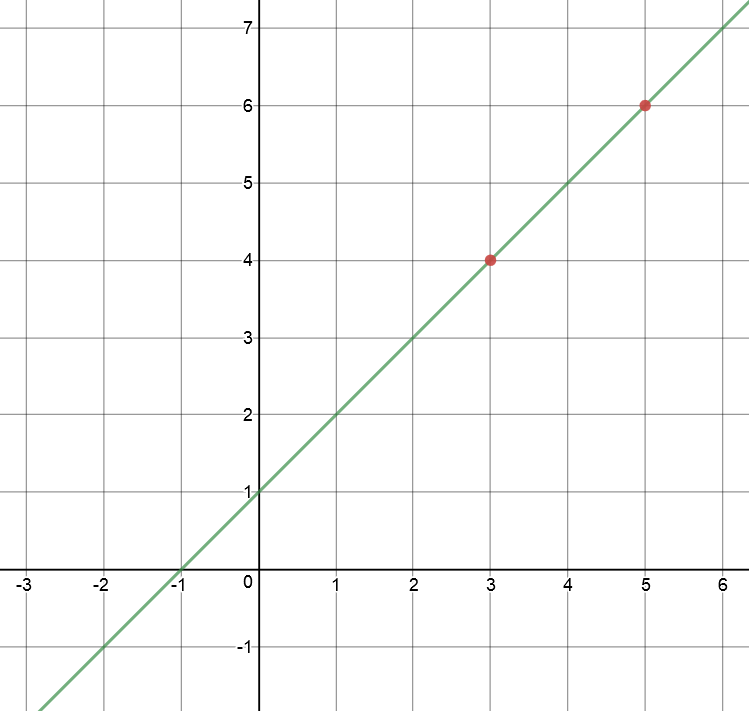To find the height of the termite mound when the base radius is 20 snouts, we plug in $\text{radius} = 20$ to give
$\text{height} = 20 + 1 = 21 \text{ snouts}.$
So, Edward's termite mound will be $21$ snouts high.

I'll leave it to you to choose the approach you wish to follow. In another article we'll show how to find the equation of a line through two points using the equation of a line through two points formula.

### Description

coordinate geometry is a branch of geometry where the position of the points on the plane is defined with the help of an ordered pair of numbers also known as coordinates. In this tutorial series, you will learn about vast range of topics such as Cartesian Coordinates, Midpoint of a Line Segment etc

### Audience

year 10 or higher, several chapters suitable for Year 8+ students.

### Learning Objectives

Explore topics related to Coordinates Geometry

Author: Subject Coach
You must be logged in as Student to ask a Question.